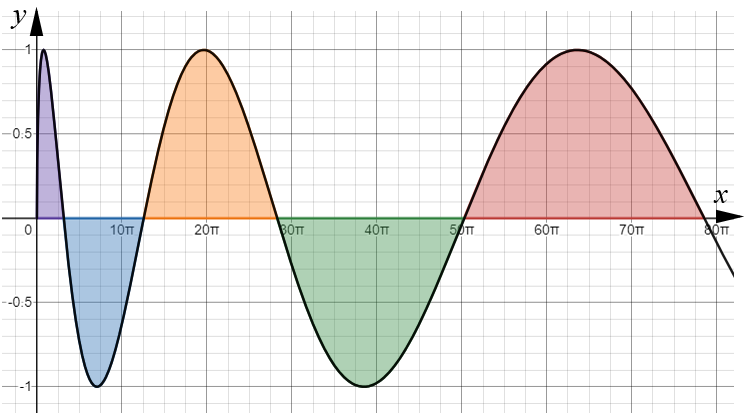# Exam-Style Question on Integration

## A mathematics exam-style question with a worked solution that can be revealed gradually

##### List Of QuestionsExam-Style QuestionMore Integration QuestionsMore on this Topic

Question id: 661. This question is similar to one that appeared on an IB AA Higher paper in 2023. The use of a calculator is allowed.

The curve $$y=\sin(\sqrt{x}) \text{ where } x \ge 0$$ intersects the x axis at the points $$x_0, x_1, x_2, x_3, x_4, ...$$ and $$x_0 = 0$$.

(a) Find $$x_1$$, the first x-intercept of the function to the right of the origin. Give your answer in terms of $$\pi$$.

(b) Find an expression for the nth x-intercept of the curve, in terms of $$\pi$$.

(c) By using an appropriate substitution, show that:

$$\int \sin(\sqrt{x}) \; dx = 2\sin(\sqrt{x}) - 2 \sqrt{x} \cos(\sqrt{x})$$

The area of the region bounded by the curve and the x-axis is denoted by $$R_n$$ where:

$$R_n = \int^{x_{n+1}}_{x_n} y \; dx$$(d) Calculate the area of region $$R_n$$ giving your answer in terms of $$\pi$$.

(e) Hence, show that the areas of the regions bounded by the curve and the x-axis, form an arithmetic sequence.

 The worked solutions to these exam-style questions are only available to those who have a Transum Subscription. Subscribers can drag down the panel to reveal the solution line by line. This is a very helpful strategy for the student who does not know how to do the question but given a clue, a peep at the beginnings of a method, they may be able to make progress themselves. This could be a great resource for a teacher using a projector or for a parent helping their child work through the solution to this question. The worked solutions also contain screen shots (where needed) of the step by step calculator procedures. A subscription also opens up the answers to all of the other online exercises, puzzles and lesson starters on Transum Mathematics and provides an ad-free browsing experience.Drag this panel down to reveal the solution

If you would like to interact with the graph mentioned in this question you will find it waiting for you on the Graph Plotter.

The exam-style questions appearing on this site are based on those set in previous examinations (or sample assessment papers for future examinations) by the major examination boards. The wording, diagrams and figures used in these questions have been changed from the originals so that students can have fresh, relevant problem solving practice even if they have previously worked through the related exam paper.

The solutions to the questions on this website are only available to those who have a Transum Subscription.

Exam-Style Questions Main Page

Search for exam-style questions containing a particular word or phrase:

To search the entire Transum website use the search box in the grey area below.